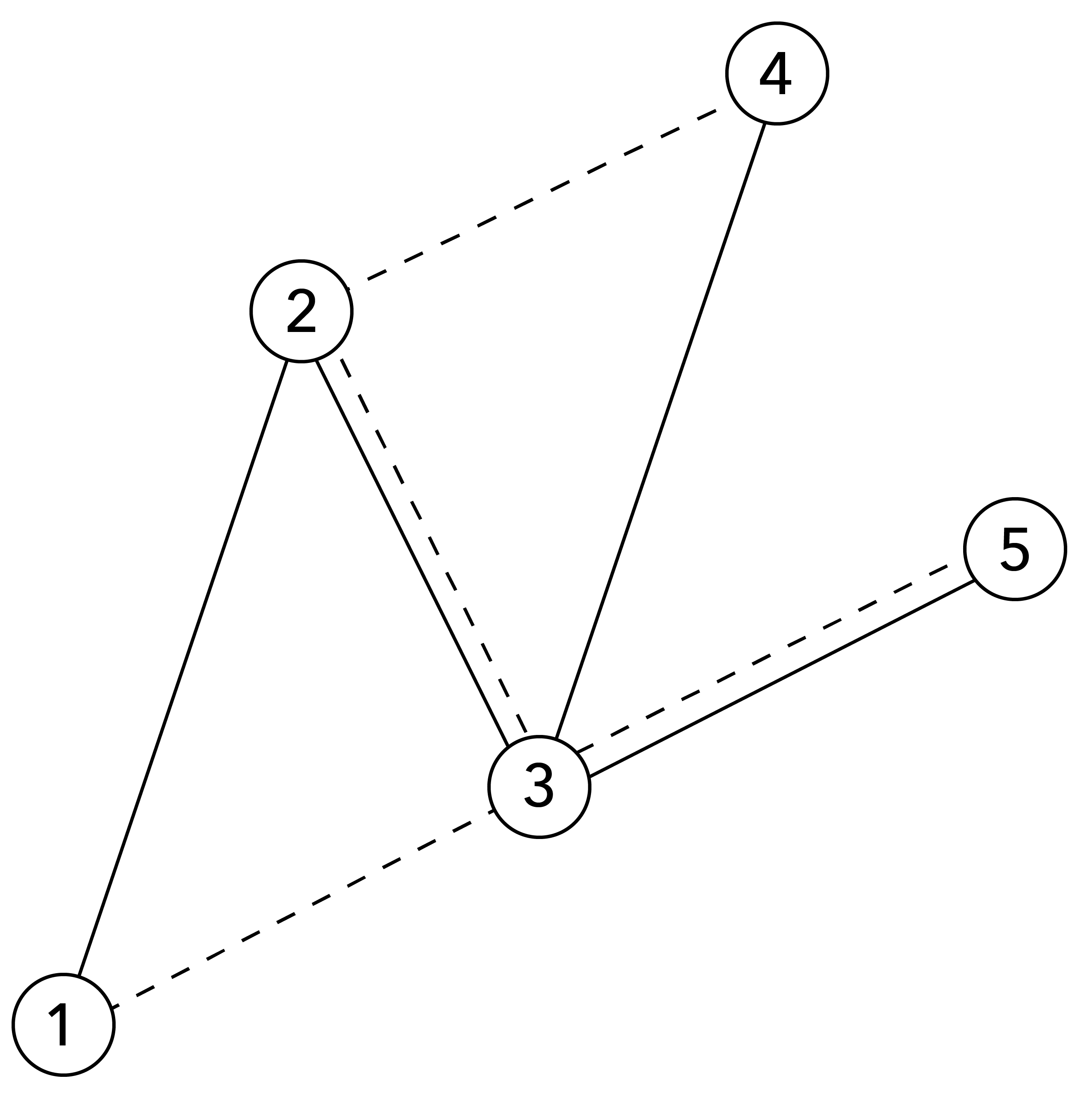시간 제한메모리 제한제출정답맞힌 사람정답 비율
2 초 (추가 시간 없음) 1024 MB66484873.846%

## 문제

Donghyun recently bought a square-shaped tablecloth. There are $N$ dots on the cloth, and the dots can be seen from both sides of the cloth. Donghyun thought that the tablecloth can be made more beautiful, so he decided to decorate the cloth with sewing.

For convenience, let's assume that each dot is a point on the $xy$-plane and the dots are numbered from $1$ to $N$. Dot $i$ ($1 \le i \le N$) is placed at coordinate $(x_i, y_i)$. No two dots have the same coordinates. A sewing sequence is an integer sequence $\{ s_i \}$ of length $k \geq 2$ satisfying $1 \le s_i \le N$ ($1 \le i \le k$) and $s_i \neq s_{i+1}$ ($1 \le i \le k-1$).
The sequence draws edges on the cloth per the following rules:

• Draw an edge connecting dot $s_{2i-1}$ and dot $s_{2i}$ on the front side of the cloth for all $1 \le i \le \left \lfloor \frac{k}{2} \right \rfloor$.
• Draw an edge connecting dot $s_{2j}$ and dot $s_{2j+1}$ on the back side of the cloth for all $1 \le j \le \left \lfloor \frac{k-1}{2} \right\rfloor$.

Donghyun wants to make a \textbf{beautiful pattern} on the tablecloth, which is defined as the following:

• For both sides of the cloth, all $N$ dots are connected by the edges on that side.
• Two edges on the same side of the cloth can intersect only at a common endpoint.

Donghyun is very busy, so he wants to finish his sewing job as quickly as possible. In other words, over all sewing sequences that produces a beautiful pattern, Donghyun decides to choose the shortest such sequence. Your job is to find such a sequence.

Note that Donghyun wants to minimize the length of the sewing sequence itself, not the sum of the lengths of the edges he draws.

## 입력

On the first line, a single integer $N$ is given. ($2 \le N \le 1\,000$)

For each of the next $N$ lines, two integers $x_i$ and $y_i$ are given, which means dot $i$ is placed at coordinate $(x_i, y_i)$. ($1 \le x_i, y_i \le 10^9$)

No two dots are at the same coordinates.

## 출력

On the first line, output a positive integer $k$, the length of the shortest sewing sequence that produces a beautiful pattern.

On the next line, output $s_1$, $s_2$, $\cdots$, $s_k$, the actual sewing sequence.

It can be proven that, for every possible input, there exists a sewing sequence that produces a beautiful pattern.

## 예제 입력 1

5
1 1
2 4
3 2
4 5
5 3


## 예제 출력 1

9
1 2 4 3 2 3 5 3 1Figure 1. Visualization of sample output. Solid lines represent for edges on front side, dashed lines represent for back side.